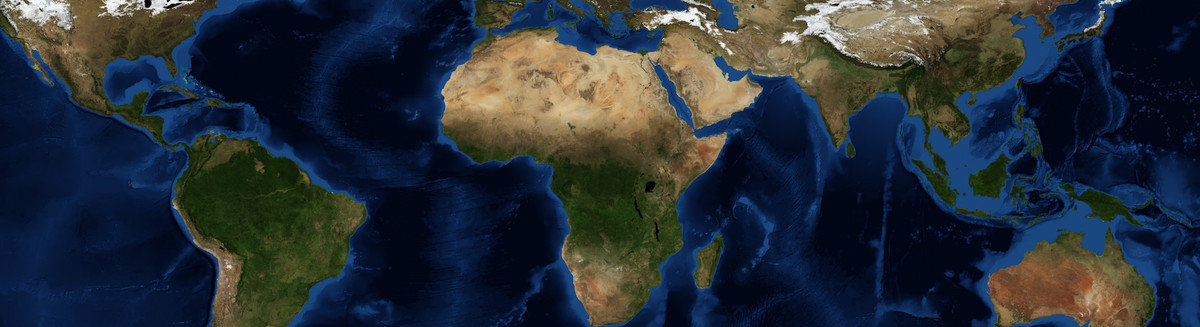This is a Preprint and has not been peer reviewed. This is version 2 of this Preprint.

##### Authors

Jean-Michel Brankart

##### Abstract

The purpose of this study is to further investigate the assumption that geophysical flows can be described and simulated by direct sampling from a dynamically constrained probability distribution, with a reversible formulation of dissipation. This is done by making the probability of the flow decrease with the deviation of entropy with respect to local equilibrium, and by assuming that this deviation can be computed from the local structure of the macroscopic flow. It is shown that this formalism can reproduce a wide variety of linear dissipation behaviours by adjusting appropriately the spectrum of the stochastic fluctuations in the macroscopic flow, and that it can be easily extended to include nonlinear dissipation in the probability distribution. This reversible, stochastic and nonlinear formulation of dissipation is then applied to the simulation of turbulent geophysical flows, using the lowest-order nonlinear term, with constant parameters. Results obtained for the turbulent boundary layer (in canal flows) show that the main observed characteristics of the flow can be quite faithfully reproduced. In particular, far enough from the boundary conditions, the universal logarithmic velocity profile is an analytic solution of the governing equation, and the behaviour of the friction coefficient as a function of the Reynolds number is correctly simulated by this single model, across the viscous and turbulent regimes. Unlike linear dissipation, the nonlinear dissipation term is specific to the reversible formulation of the problem, and has no equivalent as a forward-in-time diffusion equation. On the other hand, results obtained with a shallow-water model suggest that the same approach can be applied to more complex flows, and that it might become applicable to produce a probabilistic forecast of geophysical flows. More generally, this study suggests that it is possible to construct a reversible probabilistic dynamics that connects observations across time, at a macroscopic level, without explicitly resolving the finest structures of the system.

##### DOI

https://doi.org/10.31223/osf.io/jpg85

##### Subjects

Earth Sciences, Fluid Dynamics, Physical Sciences and Mathematics, Physics

##### Dates

Published: 2020-06-19 17:09

Last Updated: 2023-10-10 21:35